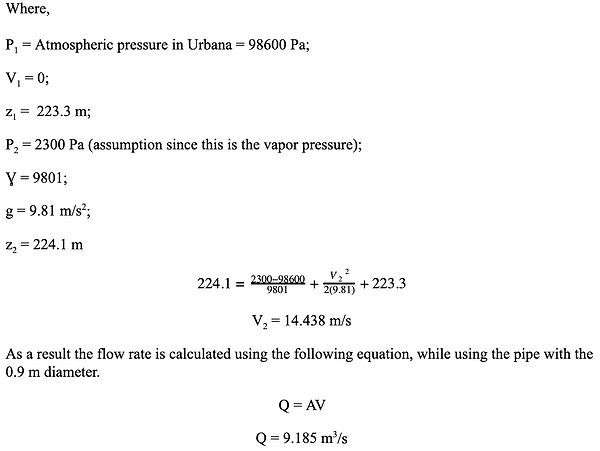top of page

### Flow-rate Calculations

In this question, absolute pressures shall be used:

Assumption: No loss in energy

As a result of the aforementioned assumption, given that the flow rate is mainly dependent on the Area of the pipe and the velocity, the flow rate would only differ if the pipe diameter would change. Also the main aim of this part is to find the critical velocity at which there would be no entrained air/at which cavitation may occur, which happens when the static pressure is less than the vapor pressure. Thus Bernoulli’s equation can be used as follows:bottom of page